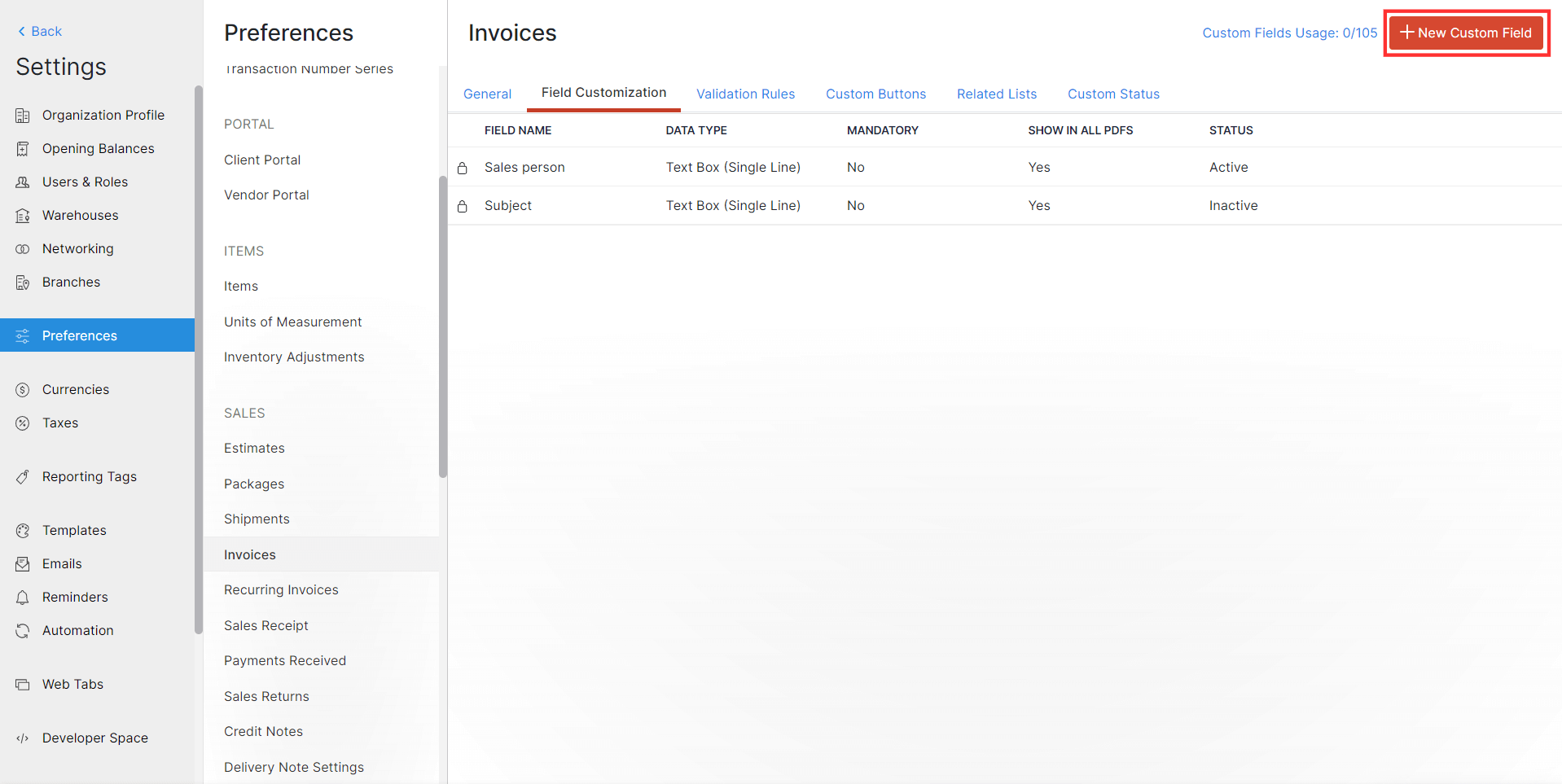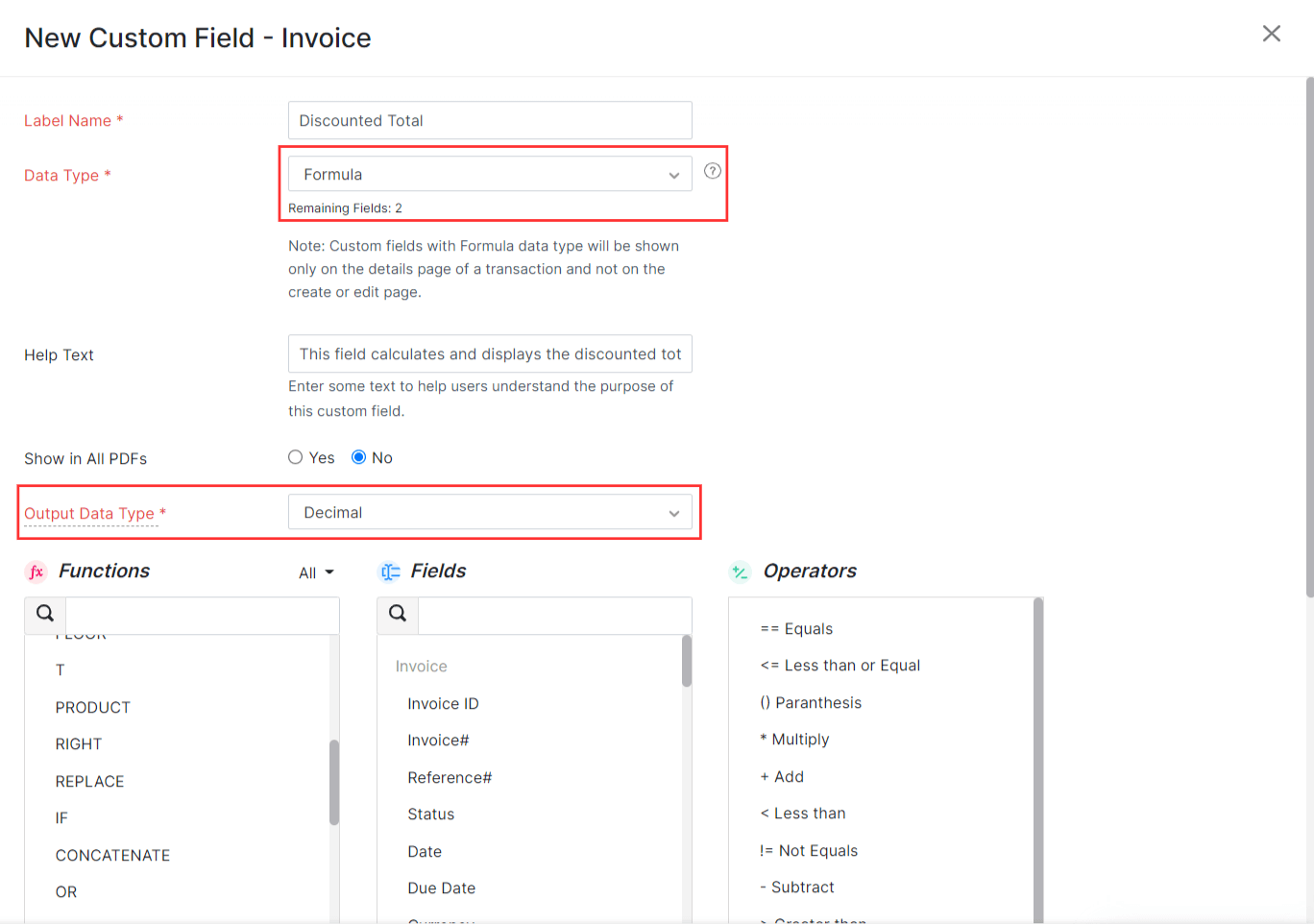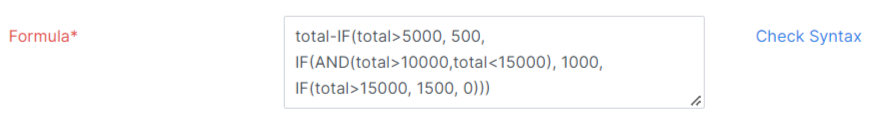# Formula Data Type

Formula data type in a custom field enables you to build a formula that performs calculations using predefined functions, fields, and operators. This formula will then be executed and the output will be displayed in the custom field. Let’s see how you can use this in Zoho Books with the help of few scenarios.

Scenario 1: Door to Door is an e-commerce company. Most of their customers have common first names. To identify their customers easily they create a custom field called Customer Identity. Customer Identity is a unique value obtained by combining the values in the Customer Name and Phone Number fields. Door to Door uses the formula data type in custom fields to combine the Customer Name and Phone Number fields, and stores the value in the Customer Identity field.

Scenario 2: Premier Courier is a courier and package distribution company operating in India. They calculate an item’s weight in kilograms. They have customers in Canada where weight is calculated in pounds. Premier Courier uses the formula data type in custom fields to convert the weight from kilogram to pound.

The formula data type consists of three elements:

• Function: A function performs a specific action based on the values that you provide as input and generates a new value as output. To create a function, enter the function name followed by a set of parenthesis. For example: Abs(), Ceiling(), etc.
• Argument: An argument is the value given within the parenthesis of a function. It can be a number, text, or other function. If there is more than one argument, you can separate them using commas. For example: Max(20, 5, 45), etc.
• Operator: An operator is the mathematical symbol using which arguments in the function perform calculations. For example: If(2==2), etc.

We will look at each of these elements in detail below.

### Functions in Formula Data Type

A function is a predefined formula that you can use to perform mathematical, text, or logical operations. To create a function, you should enter the function name followed by the required arguments inside a set of parenthesis. The functions supported by the formula data type are:

• Mathematical functions
• Text functions
• Logical functions

#### Mathematical Functions

Mathematical functions are used to perform mathematical calculations.

The following table contains the list of mathematical functions available in formula data type and examples of how they can be used.

Function Name Description Syntax Examples No. of Arguments Required Argument Data Type Output Data Type
Abs Returns the absolute value of a number. Abs(number) ABS(-42) returns 42. 1 Number Number
Addmonths Adds or subtracts the specified number of months from the given date and returns the new date. ADDMONTHS(date, number) ADDMONTHS(“2022-10-17”, 3) returns 2023-01-17, ADDMONTHS(“2022-10-17”, -3) returns 2022-07-17. 2 String, Number Date
Avg Returns the average value of the given numbers. AVG(number1, number2, …, number30) AVG(1, 2, 3) returns 6. Multiple All Number Number
Ceiling Returns the least integer greater than or equal to the number. CEILING(number) CEILING(3.4) returns 4. 1 Number Number
Date Combines the values given, converts them to the date format yyyy/mm/dd and returns the date in this format. DATE(year, month, day) DATE(2022, 10, 17) returns 2022/10/17. 3 Number, Number, Number Date
Floor Returns the greatest integer lesser than or equal to the number. FLOOR(number) FLOOR(3.8) returns 3. 1 Number Number
Max Returns the largest number from the numbers provided. MAX(number1, number2, …, number 30) MAX(3, 1, 5, 2) returns 5. Multiple All Number Number
Min Returns the smallest number from the numbers provided. MIN(number1, number2, …, number 30) MIN(5, -3, 0, 1) returns -3. Multiple All Number Number
Percentage Returns what percentage of number1 is number2. PERCENTAGE(number1, number2) PERCENTAGE(20,5) returns 25. 2 Number, Number Number
Product Multiplies the given numbers. PRODUCT(number1, number2, …, number30) PRODUCT(5, 6) returns 30. Multiple All Number Number
Round The given number is rounded up to the specified number of decimal places. ROUND(number, decimal place) ROUND(123.344, 2) returns 123.34. 2 Number, Number Number
Sum Returns the sum of all the given numbers. SUM(number1, number2, …, number 30) SUM(3, 8, 5) returns 16. Multiple All Number Number, String
Sqrt Returns the square root of the given number. SQRT(number) SQRT(9) returns 3. 1 Number Number,String

Insight: Here, the output data type string refers to Text Box (Single Line).

#### Text Functions

Text functions are used to manipulate or edit text in any form.

The following table contains the list of text functions available in formula data type and examples of how they can be used.

Function Name Description Syntax Examples No. of Arguments Required Argument Data Type Output Data Type
Char Returns the character equivalent of the given number. CHAR(number) CHAR(97) returns a. 1 Number String
Clean Removes the non-printable characters from the text and returns the printable characters. CLEAN(string) CLEAN(“H¶ello”) returns Hello. 1 String String
Code Returns the numeric value of the first character of the string. CODE(string) CODE(“Apple”) returns 65. 1 String Number
Concatenate Combines two strings into a single string. CONCATENATE(string1, string2) CONCATENATE(“FirstName”, “LastName”) returns FirstNameLastName. 2 String, String String
Exact Returns true if the two strings are identical. EXACT(string1, string 2) EXACT(“Zoho”, “Zoho”) returns true. 2 String, String Boolean
Fixed Rounds the number to the specified number of decimal places, formats the number by adding commas, and returns the result. FIXED(number, decimal_places(optional), no_commas(optional)) FIXED(12345678.4367, 3, FALSE) returns 12,345,678.437. 3 Number, Number, Boolean Number
Mid Returns the specific number of characters from the string, starting from the position that you specify up to the number of characters that you specify. MID(string, starting_position, number_of_characters) MID(“welcome”, 4, 7) returns come. 3 String, Number, Number String
Left Returns the number of characters that you specify from the left side of the string. LEFT(string) LEFT(“Apple”, 3) returns App 2 String, Number String
Len Returns the length of the given string. LEN(string) LEN(“abc”) returns 3. 1 String Number
Lower Converts the given string to lower case. LOWER(string) LOWER(APPLES) returns apples. 1 String String
Proper Converts the first letter of each word in the string to upper case. Converts any other character to lower case. PROPER(string) PROPER(zoho books) returns Zoho Books. 1 String String
Right Returns the number of characters specified from the right side of the string. RIGHT(string, number_of_characters) RIGHT(“Zoho Books, 5) returns Books. 2 String, Number String
Rept Repeats the given value a specified number of times. REPT(value, number) REPT(“A”, 6) returns AAAAAA. 2 String, Number String
Replace Replaces a part of the string with a different text. REPLACE(old_text, starting_number, number_of_characters, new_text) REPLACE(“South Africa”, 1, 5, “North”) returns North Africa. 4 String, Number, Number, String String
Search Returns the position of the first occurrence of string2 inside string1. SEARCH(string1, string2, number(optional)) SEARCH(“Elephant”, “e”, 2) returns 3. 3 String, Value, Number Number
Substitute Replaces the specified text in the string with the text you specify. SUBSTITUTE(string, old text, new text, instance_of_occurrence(optional)) SUBSTITUTE(“Dog in the wall, and Dog in the street” “Dog”, “Cat”) returns Cat in the wall, and Cat in the street. 4 String, String, String, Number String
Upper Converts the given string to upper case. UPPER(string) Upper(apples) returns APPLES. 1 String String
T Returns the text if the value provided is a text. Returns a blank space if the value provided in the string is not a text. T(string) T(“India”) returns India. 1 String String
Trim Removes the leading and trailing spaces from the string. TRIM(string) TRIM(” abcd “) returns abcd. 1 String String

Insight: Here, the output data type string refers to Text Box (Single Line).

#### Logical Functions

Logical functions return one of the two values, true or false, as output based on the values given as input.

The following table contains the list of logical functions available in formula data type and examples of how they can be used.

Function Name Description Syntax Examples No. of Arguments Required Argument Data Type Return Type
And Returns true if all the conditions are true, else it returns false. AND(condition1, condition2, …, condition30) AND(2>1, 5>3, 7<8) returns true. Multiple Boolean Boolean
Count Returns the number of numerical values present in the values provided. COUNT(value1, value2, …, value30) COUNT(10, 11.5, “string”, -55, “25”) returns 3. Multiple Generic Boolean
If Returns value1 if the condition is true, else it returns value2. IF(condition, value1, value2) IF(8>7, 1, 0) returns 1. 3 Generic, Boolean, Boolean Boolean
Isblank Returns true if no input is provided. Returns false if input is provided. ISBLANK(value) ISBLANK() returns true. 1 Generic Boolean
Isnumber Returns true if the value entered is a number. Returns false if the value entered is not a number. ISNUMBER(value) ISNUMBER(25) returns true. 1 Generic Boolean
Not Returns the logical negation. Returns false if the condition is true. Returns true if the condition is false. NOT(condition) NOT(1==1) returns false. 1 Boolean Boolean
Or Returns true if anyone of the conditions is true. Returns false if none of the conditions are true. OR(condition1, condition2, …, condition30) OR(1<2, 3>5, 7>8) returns true. Multiple Boolean Boolean

Insight: Here generic implies any data type such as numeric, string, or Boolean.

### Creating a Custom Field Using the Formula Data Type

• Go to Settings and navigate to Preferences.
• Go to the module for which you are creating the formula.
• Switch to the Field Customization tab and click + New Custom Field at the top right corner of the page.• Enter the Label Name and select Formula from the dropdown next to Data Type.

• Select the data type in which you want to store and display the output of the formula from the dropdown next to Output Data Type.• Construct the formula by clicking the + button next to the respective Functions, Fields, and Operators or by double-clicking them. You can also enter the formula in the Formula field.• After constructing the formula, click Check Syntax next to the Formula field to check if the formula that you have entered is valid.
• Click Save.

Note: Custom fields created using the formula data type will be displayed only on the module’s details page and not on the module’s creation or edit page.YesNoThank you for your feedback!